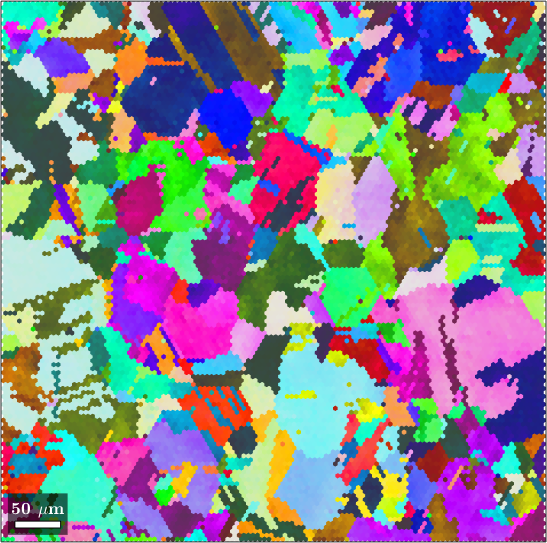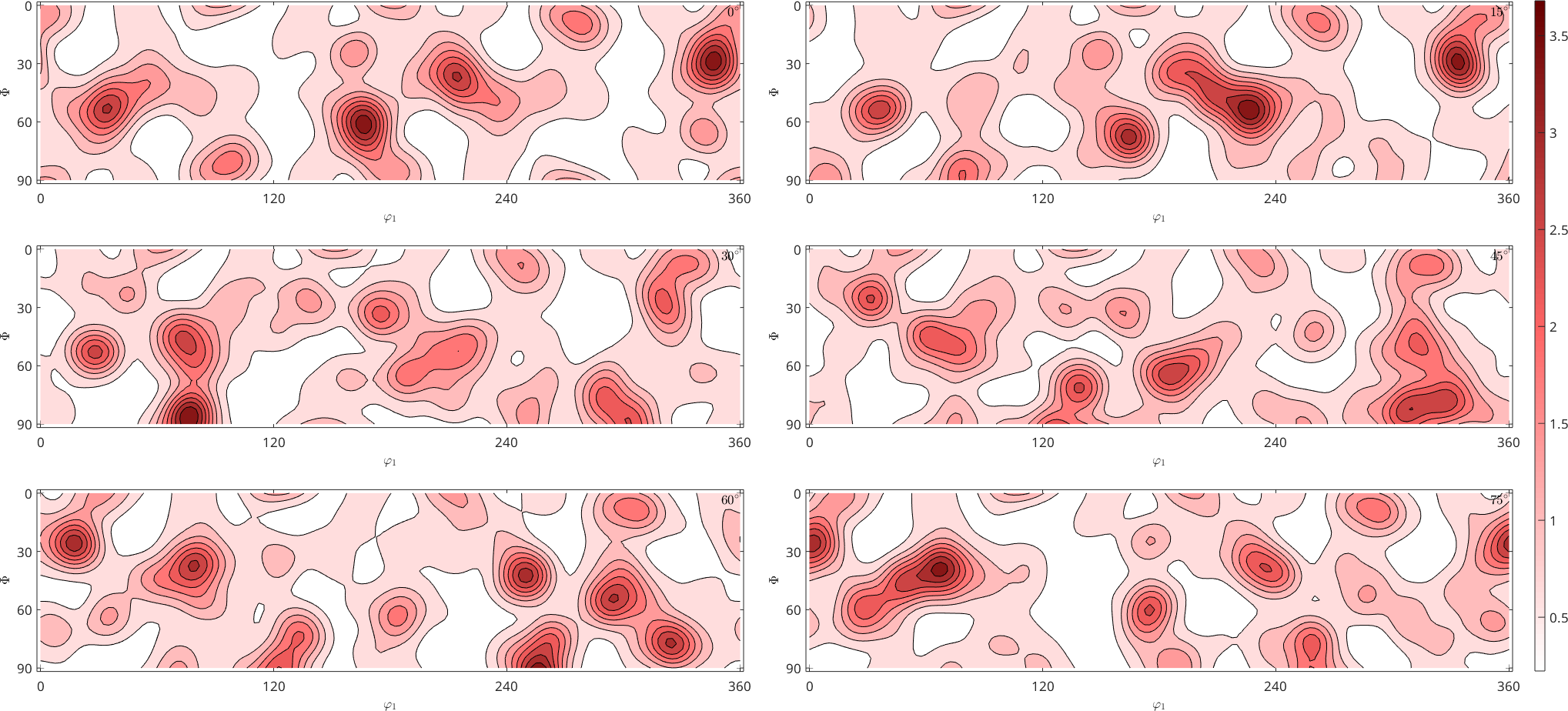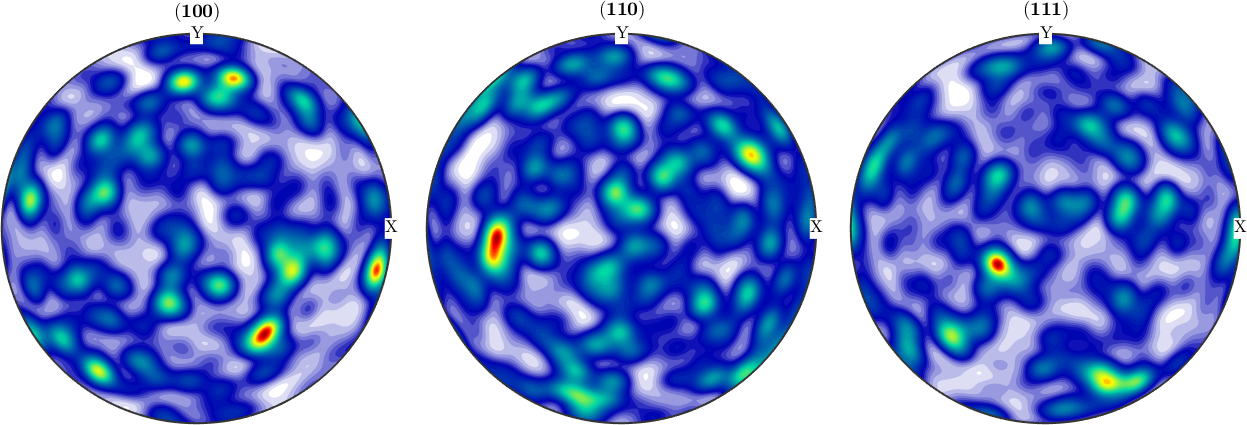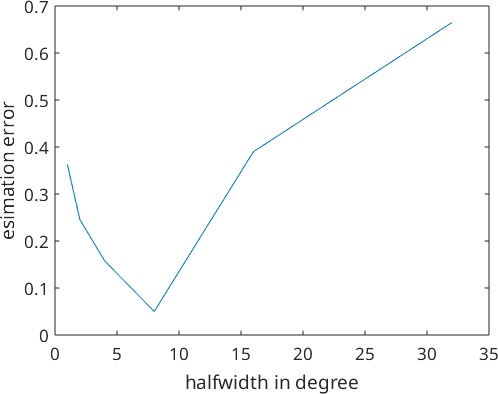ODF Estimation from EBSD data edit page

In order to discuss ODF estimation from individual orientation data we start by loading an EBSD data setof copper. The orientation distribution function can now be computed byThe function calcDensity implements the ODF estimation from EBSD data in MTEX. The underlying statistical method is called kernel density estimation, which can be seen as a generalized histogram. To be more precise, let's $$\psi : SO(3) \to R$$ be a radially symmetric, unimodal model ODF. Then the kernel density estimator for the individual orientation data $$o_1,o_2,\ldots,o_M$$ is defined as

$f(o) = \frac{1}{M} \sum_{i=1}^{M} \psi(o o_i^{-1})$

The choice of the model ODF $$\psi$$ and in particular its halfwidth has a great impact in the resulting ODF. If no halfwidth is specified the default halfwidth of 10 degrees is selected.

## Automatic halfwidth selection

MTEX includes an automatic halfwidth selection algorithm which is called by the command calcKernel. To work properly, this algorithm needs spatially independent EBSD data as in the case of this dataset of very rough EBSD measurements (only one measurement per grain).

In the above example, the EBSD measurements are spatial dependent and the resulting halfwidth is too small. To avoid this problem we have to perform grain reconstruction first and then estimate the halfwidth from the grains.

Once an ODF is estimated all the functionality MTEX offers for ODF analysis and ODF visualization is available.## Effect of halfwidth selection

As mentioned above a proper halfwidth selection is crucial for ODF estimation. The following simple numerical experiment illustrates the dependency between the kernel halfwidth and the estimated error.

Let's start with a model ODF and simulate some individual orientation data.

Next we define a list of kernel halfwidth ,

estimate for each halfwidth an ODF and compare it to the original ODF.

After visualizing the estimation error we observe that its value is large either if we choose a very small or a very large halfwidth. In this specific example, the optimal halfwidth seems to be about 4 degrees.